# Voltage Source and Current Source – Ideal vs. Practical

A source is a device which converts mechanical, chemical, thermal or some other form of energy to electrical energy. The types of sources available in the electrical network are the voltage source and the current source. The voltage source is used to provide voltage to the load while the current source is used to apply current.

## Voltage source

A voltage source is a device which provides a constant voltage to load at any instance of time and is independent of the current drawn from it. This type of source is known as an ideal voltage source. Practically, the ideal voltage source cannot be made. It has zero internal resistance. It is denoted by this symbol.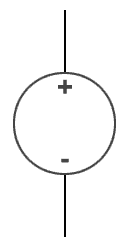Voltage source symbol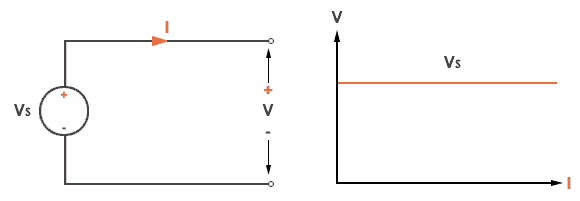Ideal Voltage Source

The graph represents the change in voltage of the voltage source with respect to time. It is constant at any instance of time.

Voltage sources that have some amount of internal resistance are known as a practical voltage source. Due to this internal resistance, voltage drop takes place. If the internal resistance is high, less voltage will be provided to load and if the internal resistance is less, the voltage source will be closer to an ideal voltage source. A practical voltage source is thus denoted by a resistance in series which represents the internal resistance of source.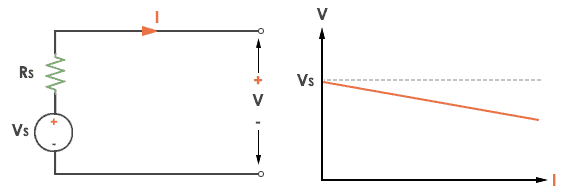Practical Voltage source

The graph represents the voltage of the voltage source with respect to time. It is not constant but it keeps on decreasing as the time passes.

## Current source

A current source is a device which provides the constant current to load at any time and is independent of the voltage supplied to the circuit. This type of current is known as an ideal current source; practically ideal current source is also not available. It has infinite resistance. It is denoted by this symbol.Current Source symbol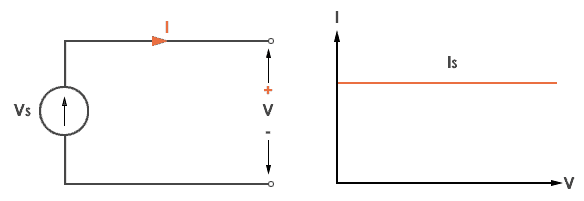Ideal Current source

The graph represents the change in current of the current source with respect to time. It is constant at any instance of time.

Why ideal Current source has infinite resistance?

A current source is used to power a load, so that load will turn on. We try to supply 100% of the power to load. For that, we connect some resistance to transfer 100% of power to load because the current always takes the path of least resistance. So, in order for current to go to the path of least resistance, we must connect resistance higher than load. This is why we have the ideal current source to have infinite internal resistance. This infinite resistance will not affect voltage sources in the circuit.

## Practical Current source

Practically current sources do not have infinite resistance across there but they have a finite internal resistance. So the current delivered by the practical current source is not constant and it is also dependent somewhat on the voltage across it.

A practical current source is represented as an ideal current source connected with resistance in parallel.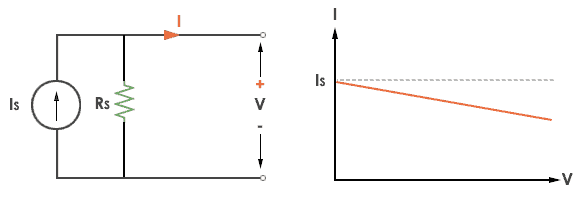Practical Current source

The graph represents the current of the current source with respect to time. It is not constant but it also keeps on decreasing as the time passes.

#### Examples of current and voltage sources

The examples of current source are solar cells, transistors and examples of some voltage sources are batteries and alternators.

This was all about ideal and practical sources of power. The ideal sources are very useful for calculations in theory but as ideal sources are not practically possible, only practical sources are used in practical circuits. The batteries we use are a practical source of power and the voltage and current decreases as we use it. Thus both are useful to us in their own ways.